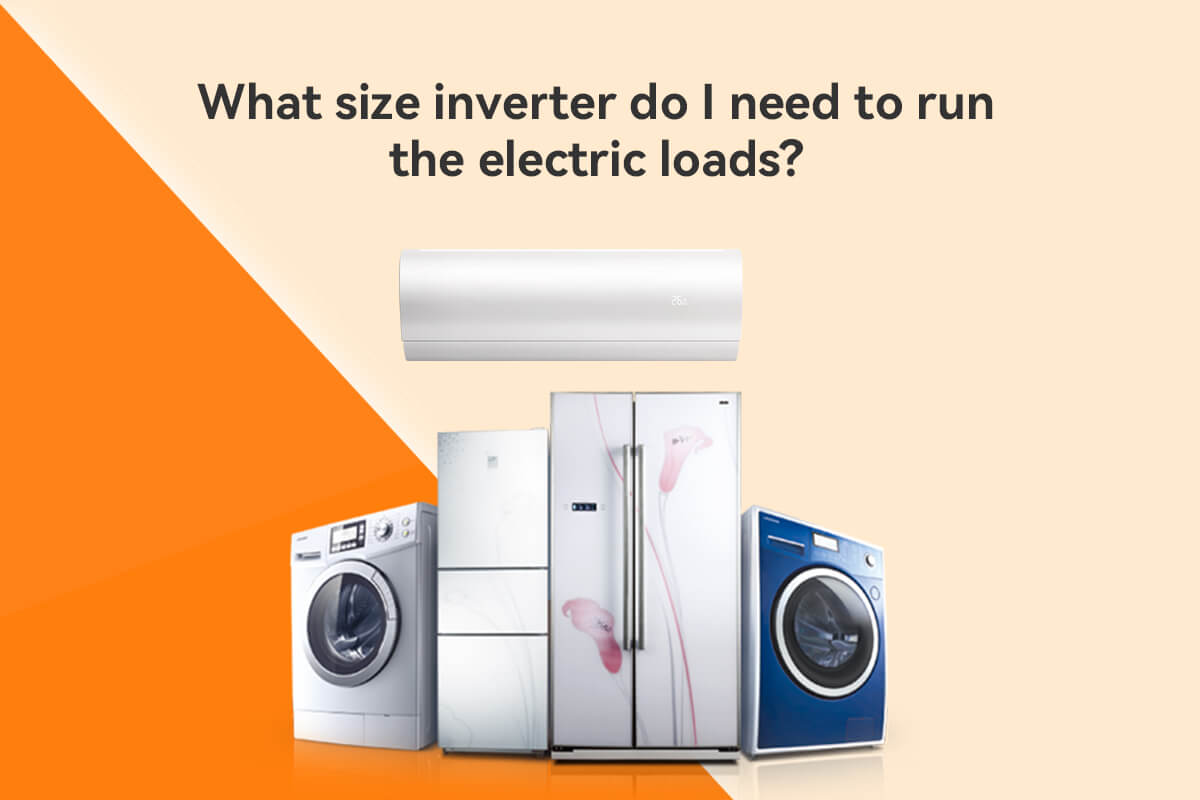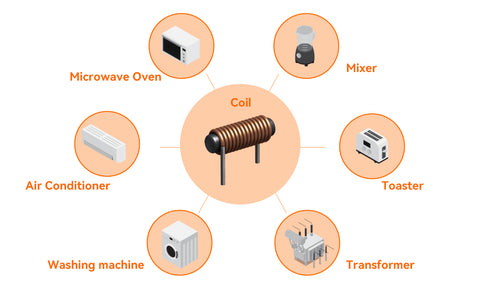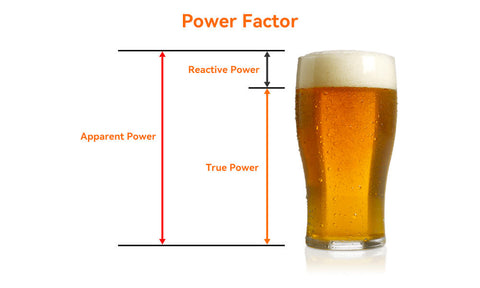# Electrical load - Determinant for inverter purchasehe solar storage inverter is the core of the PV power system. Solar panels, batteries and the grid need to rely on it to convert DC power into AC power to power the appliances, which means that the inverter is essentially serving the load. So, the load is one of the decisive factors in the specification of the whole solar storage system, and consumers would do well to have a preliminary understanding of the properties of the load before buying an inverter.

Here we introduce the concept of the three types of loads in the power system firstly.

Inductive loads are usually refers to electrical equipment containing components with coils that need to generate a magnetic field in order to function effectively, examples in everyday life are electric fans, induction cookers, air conditioners, mixers, pumps, transformers, motors, etc. Due to electromagnetic induction, the change of current is resisted and the current through the circuit lags behind the load voltage by one phase difference.In layman's terms, in the circuit, electrical equipment in which current only flows through resistive elements in the circuit is called a resistive load, common examples are incandescent lamps, electric furnaces, etc. These resistive components for work when the voltage and current in phase, that is, the phase difference is 0.

Capacitive load is generally a device whose load current exceeds the voltage by one phase difference, but, usually capacitive components do not exist independently in electrical equipment.## Why does inductive load matter?

The rated power of the loads connected to the system should be calculated and checked before purchasing the inverter. The output power of the inverter used should be determined according to the total power of the loads, taking full account of the possible impact and demand on the inverter of the surge power and reactive power required by certain special loads during start-up, and ensuring that the capacity of the inverter is large enough.

### What is true power?

In AC circuits, active power is that part of the power which is consumed in resistive elements and which cannot be reversed, e.g. into heat, light or mechanical energy.

### What is reactive power?

In electrical system, similar to inductive components made up of windings and magnets that need to generate a magnetic field in order to function, the power consumed to establish the electric field is called reactive power. In the conversion process between electrical and magnetic energy, some of the magnetic energy will return to electrical energy and no energy is consumed.

### What is apparent power?

The total power that can be supplied by an AC source is called apparent power, in numerical terms it is the product of voltage and current, in VA, apparent power is the capacity of the AC power supply.In a power system, the three types of loads introduced at the beginning of the article often exist at the same time, inductive loads are very common, but the proportion of them is different in diverse systems, the power system will also be inductive or capacitive accordingly. Under normal conditions, appliances obtain not only active power but also reactive power from the power supply.

Although reactive power is transferred back and forth between the power supply and the load without being consumed, most of the loads in the power system are inductive loads. If the reactive power provided by the inverter is insufficient when all inductive loads start at the same time, they do not have enough reactive power to establish normal electromagnetic fields, and these electric devices cannot be maintained to operate under rated conditions.

### Principle of reactive power compensation

In an AC circuit, the current in a purely resistive load is in phase with the voltage, the current in a purely inductive load lags behind the voltage, and the current in a purely capacitive load is ahead of the voltage. In other words, the phase difference between the currents in pure inductance and pure capacitance can cancel each other out.

The basic principle of reactive power compensation is to connect the device with capacitive load and inductive load in parallel in the same circuit, when the capacitive load releases energy, the inductive load absorbs energy; while the inductive load releases energy, the capacitive load absorbs energy, and energy is exchanged between the two loads.

In this way, the reactive power absorbed by the inductive load can be compensated by the reactive power output from the capacitive load, which is the basic principle of reactive power compensation.

## How to choose the inverter matching with the inductive loads

### What is power factor?

Power factor is a measure of the energy efficiency used to run machines and equipment over a certain period of time and its value is the ratio of active power to apparent power.

With a power analyzer you can measure the active power (kW) and the apparent power (kVA) of the load and thus calculate the power factor.

• PF = (True power)/(Apparent power)

When the electrical equipment is a purely resistive load or the power factor is greater than 0.9, the rated power of the inverter is selected to be 1.1 to 1.15 times the capacity of it.

### What is power surge?

A surge is a voltage rise caused by a capacitive load that will absorb part of the current when an electronic device is switched on in an electrical system. The surge capacity of the inverter (generally twice the rated power of the inverter) is a parameter you need to be aware of before buying an inverter, because when the circuit voltage exceeds this range, the electronic components of the device can be damaged.

### What is transient voltage?

Transients are generally caused by a sudden rise in voltage or current due to the release of energy stored in an inductive load. Transients are different from surges because they are more damaging and last longer. These bursts of energy can cause damage to the inverter or even other equipment.

Therefore, to avoid such failure, the consumer should ensure that the inverter power chosen is greater than or equal to the peak power of the inductive load. Generally, the peak power of inductive loads is 3 to 9 times the nominal power.

Suppose you want to buy an inverter that can instantly output 2 times the nominal power, and the capacitive or inductive load to be driven has a nominal power of 1000w, which means it has a peak power of 9000w. This peak power divided by two is the nominal power of the inverter required, i.e. 4500w.

If the power of an inverter is less than this value, two or more inverters need to be connected in parallel to provide sufficient power to the load.